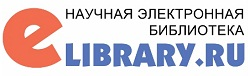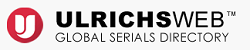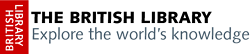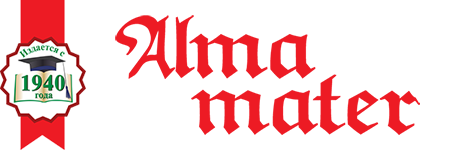ISSN 1026-955X
Vestnik Vysshey Shkoly (Higher School Herald)
The best way to learn all about Higher Education
Languages

=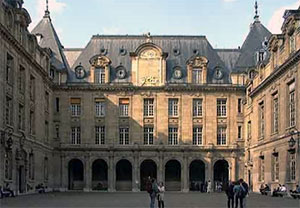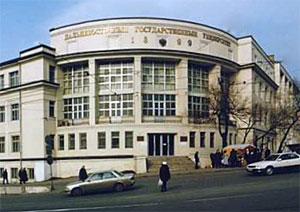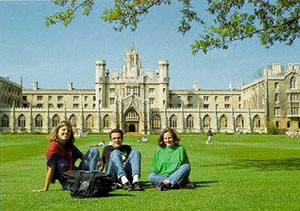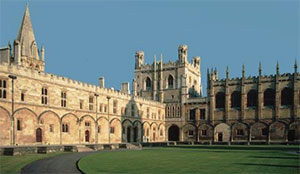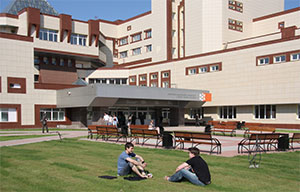# Use of differential equations in study by students of information specialties of the discipline “General theory of systems”

L.A. Sazanova
80,00 Р

https://doi.org/10.20339/AM.09-18.086

L.A. Sazanova is Cand.Sci. (Physics & Mathematics), doc. at Ural State Economical University

Analyzed is the problem of using of differential equations and their systems in the process of lecturing to students of information specialties the discipline “System theory and system analysis”, that allows applying earlier knowledge in performance of laboratory work on the topic “Population dynamics”. Substantiated is choice of this topic for teaching future computer science bachelors from the point of view of the system approach, as well as conception of inter-disciplinary connections and possibility of selection software tool, suitable for realizing calculations and experiments of program instruments. Within framework of this subject, proposed is to study models of free and limited population growth, as well as popular in the course of system analysis of the “predator-prey” model. Examples of specific tasks and tasks for laboratory work are also given.

Key words: differential equations, theory of systems, system analysis, mathematical model, dynamics of population, “predator-prey” model, difference equations, phase portrait.

References

1. Gig, J. van. Applied general theory of systems. Moscow, 1981.

2. Blauberg, I.V., Yudin, E.G. Formation and essence of systems approach. Moscow, 1973.

3. Volgina, O.A. Mathematical modeling of economic processes and systems. Moscow, 2012.

4. Ibragimov, N.Kh. Practical course of differential equations and mathematical modeling. Nizhny Novgorod, 2007.

5. Murrey, J.D. Mathematical biology: Introduction. Springer, 2002. P. 544.

6. Trubetskov, D.I. Phenomenon of mathematical model of Lotka-Volterra and similar to it. Izvestiya vysshikh uchebnykh zavedenii. 2011. No. 2. Pp. 69–88.

7. Samarskii, A.A., Gulin, A.V. Numerical methods. Moscow, 1989.

8. Ashby, U.R. Application of cybernetics in biology and sociology. Problems of philosophy. 1958. No. 12, Pp. 110–117.

9. Soetaert, K. Solving Differential Equations. Springer, 2012. P. 248.

10. Zharkova, Yu.S. Teaching elements of mathematical modeling in a pedagogical university as a means of developing professional competences. URL: https://cyberleninka.ru

ВойтиАдминистрация Волгоградской области
Комитет образования и науки Волгоградской области Выставочный центр «Царицынская ярмарка»

18-20 марта 2020 г. состоится XVI крупнейшая выставка в Волгоградской области

Волгоградский образовательный форум - 2020

Место проведения: г. Волгоград, пр. Ленина, 76, Волгоград Арена